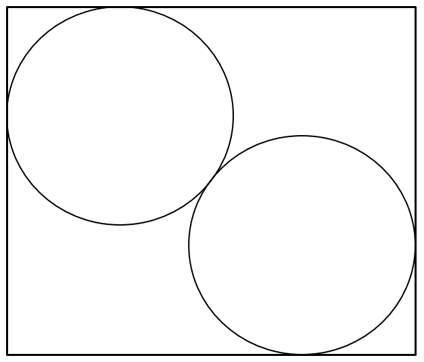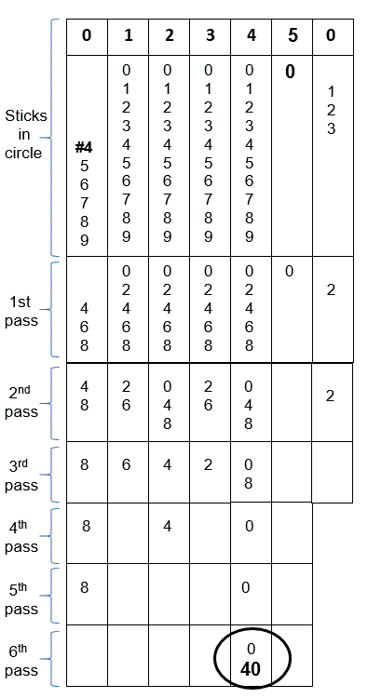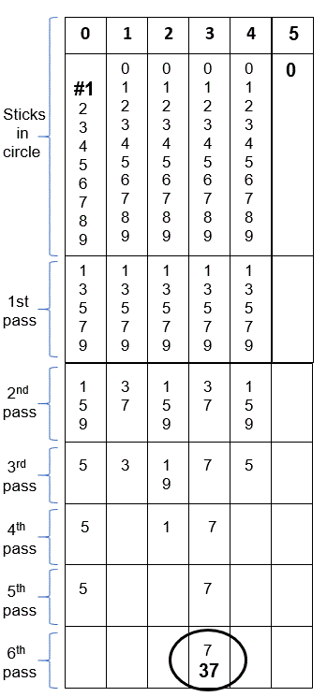Select Page

Each month, a new set of puzzles will be posted.  Come back next month for the solutions and a new set of puzzles, or subscribe to have them sent directly to you.

## MIND-Xpander Challenges

1. What would the total be if you add ‘all’ the numbers in the first 200 consecutive numbers (i.e., 1 + 2 + 3 + 4 + 5 + etc.)?
2. What would the total be if you add all the ‘even’ numbers in the first 200 consecutive numbers (i.e., 2 + 4 + 6 + 8 + 10 + etc.)?
3. What would the total be if you add all the ‘odd’ numbers in the first 200 consecutive numbers (i.e., 1 + 3 + 5 + 7 + 9 + etc.)?

## MIND-Xpander (‘Circles in a Rectangle’ Challenge – Level 1)

The diagram below shows two identical circles touching each other and completely put inside a rectangle (as shown below). The circles are not over-lapping and each touches two sides of the rectangle (as shown). What are the dimensions of the rectangle if their radius is 5cm? Note: The diagram is not drawn to scale.## Feedback

There are more than one way of doing these puzzles and may well be more than one answer.  Please let me and others know what alternatives you find by commenting below.  We also welcome general comments on the subject and any feedback you'd like to give.

If you have a question that needs a response from me or you would like to contact me privately, please use the contact form.

## Get more puzzles!

If you've enjoyed doing the puzzles, consider ordering the books;

• Book One - 150+ of the best puzzles
• Book Two - 200+ with new originals and more of your favourites

Both in a handy pocket sized format.   Click here for full details.

## RELATIONAL Challenge

In the following puzzle, there is a common arithmetic relationship between the set of equations that allow their respective solutions as shown. Determine the relationship to find the unknown relational value (?).

Solution:

1 + 4 (x1 – 0) = 5
2 + 5 (x2 – 1) = 11
3 + 6 (x3 – 2) = 19
5 + 8 (x5 – 4) = 41

## MIND-Xpander (Circular Logic Challenge – Level 1)

You have 50 sticks standing upright in a circle (#1 to #50). Starting with stick #1, knock over the next stick (#2) in the circle. Then continue by knocking over every second stick (next is #4). Keep going around and around the circle until one stick remains standing. What number is the last stick standing?

What if you start with stick #4 and continue in same manner as above?

Solution 1:                        Solution 2: